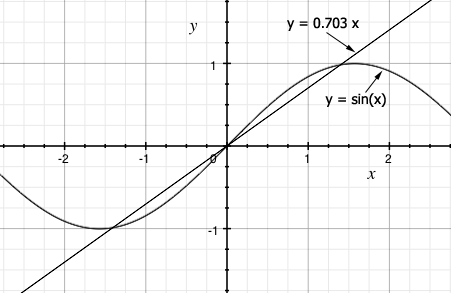SEARCH HOMEMath Central Quandaries & QueriesQuestion from Param: sinX=0.703XHi,

You didn't give instructions but I think you are to solve for $x.$ Since $\sin(x) = 0, x = 0$ is a solution. I then graphed the two functions and the graph indicates there are two other solutions also.Unfortunately there is no algebraic way to find these solutions, the best you can do is approximate them. One method of approximation is Newton's Method. You can see an example in our response to a previous question.

Penny* Registered trade mark of Imperial Oil Limited. Used under license.Math Central is supported by the University of Regina and the Imperial Oil Foundation.